hbelabs.com - personal website of Bob Jones

Thousand Digit Results

Robert Stephen Jones
rsjones7 at yahoo dot com

Some selected eigenvalues of the Laplacian $$\Delta\Psi(x,y) + \lambda\Psi(x,y) = 0$$ are calculated to a precision of at least 1000 digits, with one (regular pentagon DS-4) up to just over 2500 digits. Only Dirichlet modes ($\Psi=0$ on boundary) of the L-shape and the regular hexagon are on this list, but both Neumann ($\partial\Psi/\partial n$ on boundary) and Dirichlet modes of the regular pentagon are included.

Shape Mode Digits Date finished
Regular Pentagon DS-1 1502
2001
June 2015
October 15, 2016
Regular Pentagon DB-11000 July 6, 2016
Regular Pentagon DC-11000 August 16, 2016
Regular Pentagon DS-21001June 2016
Regular Pentagon DC-21000August 23, 2016
Regular Pentagon DB-21000 July 2016
Regular Pentagon DB-31010 August 8, 2016
Regular Pentagon DS-31000 June 2016
Regular Pentagon DC-31001September 15, 2016
Regular Pentagon DA-12002September 25, 2016
Regular Pentagon DS-42502 November 15, 2016
Regular Pentagon DS-13777 1006 June 16, 2016
Regular Pentagon NS-1 1003 November 22, 2015
Regular Pentagon NS-2 1002 November 23, 2015
Regular Pentagon NA-1 1003 November 24, 2015
Regular Pentagon NS-3 1001 November 24, 2015
Regular HexagonDS-11001 August, 2015
L-shape (matlab logo) D-11001 October 21, 2015

See below for details. Under mode, "D" or "N" means Dirichlet or Neumann (boundary conditions) and one of [ABCS] denotes the symmetry class for the regular Pentagon. The Pentagon eigenvalues are the lowest eleven in order. For example, DS-3 corresponds to the third fully-symmetric Dirichlet mode eigenvalue, but also to the ninth Dirichlet [distinct] eigenvalue. Under Regular Hexagon, DS-1 is the lowest Dirichlet (fully symmetric) mode eigenvalue.

DS-1: Lowest Dirichlet eigenvalue (first S-mode) for the regular pentagon: 2001 correct digits1500 digits: June 2015
2000 digits: October 15, 2016

In Oct 2016, I extended the DS-1 eigenvalue to 2001 correct digits. The 1502-digit bound is shown for comparison: Notice how $31460<42206.3\ldots <43652$.

The regular pentagon is inscribed in a unit-radius circle. The bound is $$\lambda=\begin{cases} 7.957089\cdots 63339\,_{31460}^{43652} & [1502\mbox{ correct digits}]\\ 7.957089\cdots 63339422063\cdots 084013683\,_{28150}^{73695}& [2001\mbox{ correct digits}] \end{cases}$$ where the first 2001 correct digits are

The $\pi$-area regular pentagon has the eigenvalue $$\lambda' = \frac{\lambda\times 5\sin(\pi/5)\cos(\pi/5)}{\pi}=6.0221379320426338\cdots$$

The unit-edged regular pentagon has the eigenvalue $$\lambda'' = \lambda\times 4\sin^2(\pi/5)= 10.996427084559806\cdots$$

DB-1: Second Dirichlet eigenvalue (first B-mode) for regular pentagon: 1000 correct digitsJuly 6, 2016

The regular pentagon is inscribed in a unit-radius circle. The bound is $$\lambda = 20.106281644908431169\cdots 53078148508016050\,_{45532}^{53575}$$ where the first 1000 correct digits are

The $\pi$-area regular pentagon has the eigenvalue $$\lambda' = \frac{\lambda\times 5\sin(\pi/5)\cos(\pi/5)}{\pi}=15.21697136244373548414\cdots$$

The unit-edged regular pentagon has the eigenvalue $$\lambda'' = \lambda\times 4\sin^2(\pi/5)= 27.78619784588530770695\cdots$$

Ratio of Eigenvalues

For the curious, the ratio of eigenvalues for DB-1 and DS-1 is about 2.52683.... This number is the ratio between the first two Dirichlet eigenvalues. It is independent of the size of the pentagon. For the equilateral triangle and the square, this ratio $\lambda_2/\lambda_1$ is rational, and for the circle it is $({j_{1,1}}/{j_{0,1}})^2 = 2.538733...$.

For the regular pentagon, the ratio is apparently a transcendental irrational, which may have been conjectured before (or was assumed to be too obvious to state), but this is the first numerical evidence at 1000-digits of precision of such an assertion.

More precisely, the bound on the ratio is obtained using $$\frac{\lambda_2(\mbox{lower})}{\lambda_1(\mbox{upper})} \lt \frac{\lambda_2}{\lambda_1} \lt \frac{\lambda_2(\mbox{upper})}{\lambda_1(\mbox{lower})}$$ and then rounding the lower bound down and the upper bound up. The bound is found to be $$\frac{\lambda_2}{\lambda_1} = 2.52683872972705709678\cdots 960953111962085^{63141}_{52780}$$ where the first 1000 correct digits are

Continued Fraction: The continued fraction that accurately reproduces those 1000 digits in the ratio is $$\frac{\lambda_2}{\lambda_1}=\fbox{2}+\frac{1}{\fbox{1}+\frac{1}{\fbox{1}+\frac{1}{\fbox{8}+\frac{1}{...}}}}$$

If the last one or two terms are dropped from the continued fraction expansion, then it changes the trailing digit of the ratio. In other words, this is the smallest continued fraction in this format that will reproduce the ratio to 1000 digits.

Base-$\varphi$: In base $\varphi$, the golden ratio $\varphi=1+1/\varphi=\frac12\left(1+\sqrt{5}\right)=1.6180339887\cdots$, the ratio of eigenvalues is expressed in standard form $$\frac{\lambda_2}{\lambda_1}= 10.10100010101010\cdots 00000101010010100_\varphi$$ where first 4775 phigits (whimsical term phi-digits, as bit is derived from binary-digit) determined to be

[download] Like the continued fraction, the number of phigits is determined by varying the numerical value of $\lambda_2$ in the right-most least significant digit to experimentally determine how this representation varies. In effect, this is the fewest number of phigits (in standard form) that will reproduce the decimal ratio.

DC-1: Third Dirichlet eigenvalue (first C-mode) for the regular pentagon: 1000 correct digitsAugust 16, 2016

The regular pentagon is inscribed in a unit-radius circle. The bound is $$\lambda = 35.654694217826827462\cdots 33667309375148828\,_{59232}^{70636}$$ where the first 1000 correct digits are

The $\pi$-area regular pentagon has the eigenvalue $$\lambda' = \frac{\lambda\times 5\sin(\pi/5)\cos(\pi/5)}{\pi}=26.98442558556082579186\cdots$$

The unit-edged regular pentagon has the eigenvalue $$\lambda'' = \lambda\times 4\sin^2(\pi/5)= 49.27357555055232854621\cdots$$

DS-2: Fourth Dirichlet eigenvalue (second S-mode) for the regular pentagon: 1001 correct digitsJune 2016

The regular pentagon is inscribed in a unit-radius circle. The bound is $$\lambda = 41.313935\cdots 12662\,_{12604}^{68918}$$ where the first 1001 correct digits are

The $\pi$-area regular pentagon has the eigenvalue $$\lambda' = \frac{\lambda\times 5\sin(\pi/5)\cos(\pi/5)}{\pi} = 31.2674908884708557\cdots$$

The unit-edged regular pentagon has the eigenvalue $$\lambda'' = \lambda\times 4\sin^2(\pi/5) = 57.0944550842568998\cdots$$

Ratio of Eigenvalues

For the curious, the ratio of eigenvalues for DS-2 and DS-1 is about 5.19209.... This is independent of the size of the pentagon. More precisely, this ratio is (presumably to about 1000 precise digits):

The continued fraction that accurately reproduces those 1000 digits is $$\lambda=\fbox{5}+\frac{1}{\fbox{5}+\frac{1}{\fbox{4}+\frac{1}{\fbox{1}+\frac{1}{...}}}}$$

If the last one or two terms are dropped from the continued fraction expansion, then it changes the trailing digit of the ratio. In other words, this is the smallest continued fraction in this format that will reproduce the ratio to 1000 digits.

DC-2: Fifth Dirichlet eigenvalue (second C-mode) for the regular pentagon: 1000 correct digits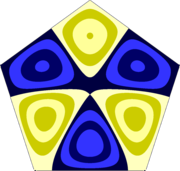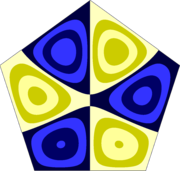August 23, 2016

The regular pentagon is inscribed in a unit-radius circle. The bound is $$\lambda = 55.700798288207054532\cdots 44314122214767707\,_{56084}^{96278}$$ where the first 1000 correct digits are

The $\pi$-area regular pentagon has the eigenvalue $$\lambda' = \frac{\lambda\times 5\sin(\pi/5)\cos(\pi/5)}{\pi}=42.15585295113685124032\cdots$$

The unit-edged regular pentagon has the eigenvalue $$\lambda'' = \lambda\times 4\sin^2(\pi/5)= 76.97661003380018810496\cdots$$

DB-2: Sixth (6th) Dirichlet eigenvalue (second B-mode) for the regular pentagon: 1000 correct digits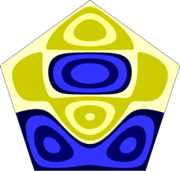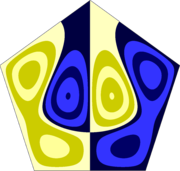The regular pentagon is inscribed in a unit-radius circle. The bound is $$\lambda = 64.521882950899216716\cdots 28966835237900411\,_{27762}^{81618}$$ where the first 1000 correct digits are

The $\pi$-area regular pentagon has the eigenvalue $$\lambda' = \frac{\lambda\times 5\sin(\pi/5)\cos(\pi/5)}{\pi}=48.83188559946443347115\cdots$$

The unit-edged regular pentagon has the eigenvalue $$\lambda'' = \lambda\times 4\sin^2(\pi/5)= 89.16704922000035471847\cdots$$

DB-3: Seventh Dirichlet eigenvalue (third B-mode) for the regular pentagon: 1010 correct digits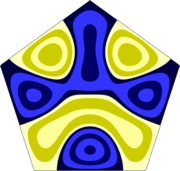August 8, 2016

The regular pentagon is inscribed in a unit-radius circle. The bound is $$\lambda = 80.406873507295220925\cdots 35700376374886065\,^{40297}_{03828}$$ where the first 1010 correct digits are

The $\pi$-area regular pentagon has the eigenvalue $$\lambda' = \frac{\lambda\times 5\sin(\pi/5)\cos(\pi/5)}{\pi}=60.85407103674935087387\cdots$$

The unit-edged regular pentagon has the eigenvalue $$\lambda'' = \lambda\times 4\sin^2(\pi/5)= 111.11956625796852916580\cdots$$

For this eigenvalue, I chose to check the alternation as $N$ is incremented for results just above the 1000-digit eigenvalue result. It took a few extra weeks of CPU, but I use this to illustrate both the global and the local convergence rate, and to numerically "verify" the bounding method. I incremented $N$ by one (two extra matching points on each iteration) after I reached 1000 eigenvalue digits, and stopped when it reached 1010 digits. Each iteration added very close to one digit, and each iteration took about two days of elapsed time.

Using the N=1134 and 1135 results yields 1000 correct digits, i.e., $80.406873\cdots 37$. The matrix size to get these results was 2Nx2N, which for N=1135 is $2270\times 2270$. In this calculation, I also used a precision of "round(2.2*N)", of close to 2500 digits, which means that each matrix element was calculated to 2500 digits of precision and so was its determinant.

The "$\pm$" represents the change in the calculated approximate eigenvalue relative to the one before it, i.e., $\mbox{sign}(\lambda_N-\lambda_{N-1})$. The alternating signs make it easy to identify the bounds. The "UB" and "LB" represent "upper bound" and "lower bound", respectively. The logarithm is approximately the number of correct digits. The actual correct digits can simply be counted.

Notice how each time, the indicated bound very nicely brackets the true eigenvalue $$\lambda=80.406873\cdots 376374886065\cdots$$ (The next digit after the 6064 is 0,1,2,3, or 4.)

The local convergence rate at 1000 digits is about $$\rho_{\rm local} = \frac{9.59}{22} = \frac{1\,{\rm digit}}{2.29\,{\rm matching\ points}}$$

The global convergence rate at 1000 digits is very close at about $$\rho_{\rm global} = \frac{1000}{2270} = \frac{1\,{\rm digit}}{2.27\,{\rm matching\ points}}$$

N$\lambda_N$ $\pm$?B$-\log_{10}\left|\frac{\lambda_N-\lambda_{N-1}|}{\lambda_N}\right|$bound
1134 $80.406873\cdots 375106074\cdots$  LB
1135 $80.406873\cdots 376545106\cdots$ $+$ UB 1000.75 $\cdots 37\,_{5106}^{6546}$
1136 $80.406873\cdots 3763520495\cdots$ $-$ LB 1001.62 $\cdots 376\,_{3520}^{5452}$
1137 $80.406873\cdots 37637794977\cdots$ $+$ UB 1002.49 $\cdots 3763\,_{5204}^{7795}$
1138 $80.406873\cdots 376374475041\cdots$ $-$ LB 1003.36 $\cdots 37637\,_{4475}^{7949}$
1139 $80.406873\cdots 3763749412077\cdots$ $+$ UB 1004.24 $\cdots 376374\,_{4750}^{9413}$
1140 $80.406873\cdots 37637487866717\cdots$ $-$ LB 1005.11 $\cdots 376374\,_{8786}^{9413}$
1141 $80.406873\cdots 376374887057584\cdots$ $+$ UB 1005.98 $\cdots 3763748\,_{7866}^{8706}$
1142 $80.406873\cdots 376374885931926\cdots$ $-$ LB 1006.85 $\cdots 37637488\,_{5931}^{7058}$
1143 $80.406873\cdots 37637488 60829455\cdots$ $+$ UB 1007.73 $\cdots 37637488\,_{5931}^{6083}$
1144 $80.406873\cdots 3763748860 6268475\cdots$ $-$ LB 1008.60 $\cdots 3763748860\,_{6268}^{8295}$
1145 $80.406873\cdots 37637488606 5402961\cdots$ $+$ UB 1009.47 $\cdots 37637488606\,_{2684}^{5403}$
1146 $80.406873\cdots 376374886065 0382826\cdots$ $-$ LB 1010.34 $\cdots 376374886065\,_{0382}^{4030}$

DS-3: Eighth (8th) Dirichlet eigenvalue (third S-mode) for the regular pentagon: 1000 correct digitsJune 2016

The regular pentagon is inscribed in a unit-radius circle. The bound is $$\lambda = 91.431308\cdots 27325\,_{05438}^{20015}$$ where the first 1000 correct digits are

The $\pi$-area regular pentagon has the eigenvalue $$\lambda' = \frac{\lambda\times 5\sin(\pi/5)\cos(\pi/5)}{\pi} = 69.1976581938886367\cdots$$

The unit-edged regular pentagon has the eigenvalue $$\lambda'' = \lambda\times 4\sin^2(\pi/5) = 126.3549608690699506\cdots$$

Ratio of Eigenvalues

For the curious, the ratio of eigenvalues for DS-3 and DS-1 is about 11.49054.... This is independent of the size of the pentagon. More precisely, this ratio is (presumably to about 1000 precise digits):

The continued fraction that accurately reproduces those 1000 digits is $$\lambda=\fbox{11}+\frac{1}{\fbox{2}+\frac{1}{\fbox{25}+\frac{1}{\fbox{1}+\frac{1}{...}}}}$$

If the last one or two terms are dropped from the continued fraction expansion, then it changes the trailing digit of the ratio. In other words, this is the smallest continued fraction in this format that will reproduce the ratio to 1000 digits.

DC-3: Ninth (9th) Dirichlet eigenvalue (third C-mode) for the regular pentagon: 1001 correct digitsSeptember 15, 2016

The regular pentagon is inscribed in a unit-radius circle. The bound is $$\lambda = 94.163411979132222848\cdots 30091133527438065\,_{04548}^{19171}$$ where the first 1001 correct digits are

The $\pi$-area regular pentagon has the eigenvalue $$\lambda' = \frac{\lambda\times 5\sin(\pi/5)\cos(\pi/5)}{\pi}= 71.26538704580909157\cdots$$

The unit-edged regular pentagon has the eigenvalue $$\lambda'' = \lambda\times 4\sin^2(\pi/5)= 130.13063485850172769\cdots$$

DA-1: Tenth (10th) Dirichlet eigenvalue (first A-mode) for the regular pentagon: 2002 correct digitsSeptember 25, 2016

The regular pentagon is inscribed in a unit-radius circle. The bound is $$\lambda = 106.47245539625886985\cdots 44665185159302310\,_{04557}^{20371}$$ where the first 2002 correct digits are

The $\pi$-area regular pentagon has the eigenvalue $$\lambda' = \frac{\lambda\times 5\sin(\pi/5)\cos(\pi/5)}{\pi}= 80.58120010788886555\cdots$$

The unit-edged regular pentagon has the eigenvalue $$\lambda'' = \lambda\times 4\sin^2(\pi/5)= 147.14131449197260431\cdots$$

DS-4: Eleventh (11th) Dirichlet eigenvalue (fourth S-mode) for the regular pentagon: 2502 correct digitsNovember 15, 2016

The regular pentagon is inscribed in a unit-radius circle. The bound is $$\lambda = 113.22322\cdots 92189\,_{83626}^{91712}$$ where the first 2502 correct digits are

The $\pi$-area regular pentagon has the eigenvalue $$\lambda' = \frac{\lambda\times 5\sin(\pi/5)\cos(\pi/5)}{\pi} = 85.6903631159731626\cdots$$

The unit-edged regular pentagon has the eigenvalue $$\lambda'' = \lambda\times 4\sin^2(\pi/5) = 156.4706488771238929\cdots$$

DS-13777: Medium-high fully symmetric Dirichlet eigenvalue for the regular pentagon: 1006 correct digits

June 16, 2016

I'm pretty sure (I need to double check) that this is the 13,777th Dirichlet eigenvalue in this (fully symmetric) symmetry class.

This eigenvalue is the first DS-eigenvalue in the unit-edged pentagon that lies above $\lambda\gt 10^6$. In this sized pentagon, it has a wavelength of about 0.00628, which corresponds to 160 wavelengths along one edge of the pentagon or about 500 wavelengths across the pentagon. To calculate it, the global convergence rate was very good at about $\rho=D/N\approx 1/1.16$. But, I had to start at a relatively large N-value of about 300 to get it (and an initial bound of $1000125.1\lt \lambda_{13777}\lt 1000125.3$).

The regular pentagon is inscribed in a unit-radius circle. The bound is $$\lambda = 723697.3815439870978979961003\cdots 17175129943 479847\,_{79047}^{83574}$$ where the first 1006 correct digits are

The $\pi$-area regular pentagon has the eigenvalue $$\lambda' = \frac{\lambda\times 5\sin(\pi/5)\cos(\pi/5)}{\pi} = 547713.5217997451217150367\cdots$$

The unit-edged regular pentagon has the eigenvalue $$\lambda'' = \lambda\times 4\sin^2(\pi/5) = 1000125.18372448931418256208721170023111247779\cdots$$

NS-1: Third (3rd) Neumann eigenvalue (first S-mode) for the regular pentagon: 1003 correct digits

November 22, 2016 (completed in 9.0 hrs, i7/6-core/64GB RAM)

The regular pentagon is inscribed in a unit-radius circle. (Disregard the trivial mode with $\lambda=0$ and a constant eigenfunction.) The bound of this eigenvalue is $$\lambda = 18.712058 \cdots 46836\,_{19363}^{20122}$$ where the first 1003 correct digits are

The $\pi$-area regular pentagon has the eigenvalue $$\lambda' = \frac{\lambda\times 5\sin(\pi/5)\cos(\pi/5)}{\pi} = 14.1617858576649162\cdots$$

The unit-edged regular pentagon has the eigenvalue $$\lambda'' = \lambda\times 4\sin^2(\pi/5) = 25.85942854984412015\cdots$$

NS-2: Seventh (7th) Neumann eigenvalue (second S-mode) for the regular pentagon: 1002 correct digits

November 23, 2016 (completed in 9.0 hrs, i7/6-core/64GB RAM)

The regular pentagon is inscribed in a unit-radius circle. (Disregard the trivial mode with $\lambda=0$ and a constant eigenfunction.) The bound of this eigenvalue is $$\lambda = 44.914021 \cdots 387523\,_{07770}^{53983}$$ where the first 1002 correct digits are

[download] $$\lambda' = \frac{\lambda\times 5\sin(\pi/5)\cos(\pi/5)}{\pi} = 33.9921322523126656\cdots$$

The unit-edged regular pentagon has the eigenvalue $$\lambda'' = \lambda\times 4\sin^2(\pi/5) = 62.06965167177503800\cdots$$

NA-1: Eleventh (11th) Neumann eigenvalue (first A-mode) for the regular pentagon: 1003 correct digits

November 24, 2016 (jupiter, ?? hrs, i7,4-core,16GB RAM)

The regular pentagon is inscribed in a unit-radius circle. (Disregard the trivial mode with $\lambda=0$ and a constant eigenfunction.) The bound of this eigenvalue is $$\lambda = 58.336226931\cdots 14894\,_{68282}^{70198}$$ where the first 1003 correct digits are

[download] Inscribe in unit-radius circle: 58.33622693119616455048 Pi Area regular pentagon: unit-edged regular pentagon: $$\lambda' = \frac{\lambda\times 5\sin(\pi/5)\cos(\pi/5)}{\pi} = 44.1504157895761852\cdots$$

The unit-edged regular pentagon has the eigenvalue $$\lambda'' = \lambda\times 4\sin^2(\pi/5) = 80.6186828434861258\cdots$$

NS-3: Eleventh (11th) Neumann eigenvalue (third S-mode) for the regular pentagon: 1001 correct digits

November 24, 2016

The regular pentagon is inscribed in a unit-radius circle. (Disregard the trivial mode with $\lambda=0$ and a constant eigenfunction.) The bound of this eigenvalue is $$\lambda = 71.611200 \cdots 51120\,_{28564}^{64868}$$ where the first 1001 correct digits are

[download] $$\lambda' = \frac{\lambda\times 5\sin(\pi/5)\cos(\pi/5)}{\pi} = 54.1972709080045915\cdots$$

The unit-edged regular pentagon has the eigenvalue $$\lambda'' = \lambda\times 4\sin^2(\pi/5) = 98.9642456628121825\cdots$$

Lowest Dirichlet eigenvalue for the regular hexagon: 1001 correct digits

August 2015

The regular hexagon is inscribed in a unit-radius circle, which is (for this shape only) the same as a unit-edged regular hexagon. The bound is $$\lambda = 7.155339\cdots 28158\,_{08891}^{15027}$$ where the first 1001 correct digits are

The $\pi$-area regular hexagon has the eigenvalue $$\lambda' = \frac{\lambda\times 6\sin(\pi/6)\cos(\pi/6)}{\pi}\approx 5.9174178316136612\cdots$$

Lowest Dirichlet eigenvalue for the L-shape: 1001 correct digits

October 21, 2015

The L-shape is formed by joining two unit-edged squares to adjacent edges of a third. The eigenfunction of this lowest Dirichlet eigenvalue is used to construct the famous matlab logo. The bound is $$\lambda = 9.6397238440219410527\cdots 27494610057016061858\,_{4831}^{5300}$$ where the first 1001 correct digits are

Note on Convergence Rate:

If $N>65$ is the number of matching points, and $D$ the number of correct digits, $$D \approx (0.4722) N + (11.7)$$ or equivalently $$N \approx (2.1177) D - (24.8)$$ indicates the approximate convergence rate. Of course, it becomes more CPU time and memory consuming as $N$ is incremented. At each of the indicated $N$ values, at least eight point-matching matrices and their determinants were calculated, four for $N$ and four for $N-2$.

Number of matching points $N$, resulting correct digits $D$, finish time (2015, CST).
Started 10/2 23:33:36
with $N=66$.
$N$ $D$ Finish Time
68 41 10/2 23:33:48
90 53 10/2 23:33:57
112 64 10/2 23:34:17
164 89 10/2 23:35:05
216 113 10/2 23:37:23
318 163 10/2 23:44:42
420 212 10/3 00:26:18
722 355 10/3 04:00:33
1024 497 10/3 15:47:06
1326 639 10/4 21:49:45
1628 780 10/6 12:14:29
1930 922 10/12 21:40:32
2098 1001 10/21 16:31:47

Some Notes

August 20, 2016: Bunimovich mushroom 100-digit results

August 8, 2016: I have been focusing on the regular pentagon. Indeed, for the last few months, I have been calculating the lowest few Dirichlet eigenvalues, each to at least a thousand digits. But I do have results for a medium high regular pentagon (500 wavelengths across the pentagon, but calculated within the triangular fundamental region), the lowest L-shape (using the fundamental region of the square), and the lowest regular hexagon (using its triangular fundamental region) ... all Dirichlet eigenvalues.

Earlier notes:

Note on Difficulty: The antisymmetric regular polygon modes are easier to calculate since the point-matched edge is odd; while the symmetric modes have an even point-matched edge. The B and C modes are calculated in a kite-shaped region, twice as large, and one point-matched edge is even, so they are more difficult than either the A or S modes. By "more difficult", I simply mean that it requires more computing power for a given precision. Corresponding Neumann and Dirichlet modes have similar levels of difficulty since the regular polygon boundary condition is enforced with a straightforward sine or cosine using the basis functions.

Higher eigenvalues get progressively more difficult to calculate using this method since it must start with correspondingly higher $N\mbox{-values}$ so that the largest gap between matching points is somewhat smaller than distance between nodes of a free wave of the same frequency. For reference, the free wavelength is $\Lambda=2\pi/\sqrt{\lambda}$, where $\lambda$ is the eigenvalue; and the point matched edge is an apothem for A and S modes, and effectively two apothems for B and C modes.

The lowest Neumann mode is in the B symmetry class while the lowest Dirichlet is in the S symmetry class. I chose the below set of eigenvalues because they are reasonably difficult and perhaps interesting.

More Questions: Very little is known about such non-closed-form eigenvalues for any shape since these are the only such high precision eigenvalues - of at least 1000 digits - that have been calculated thus far. So, interesting questions arise: Is there a relation between them? Or between them and $\pi$? Are they transcendental irrationals? Are these eigenvalues normal numbers (we don't even know if $\pi$ is, and over 12 trillion of its digits are known)? etc. Enjoy.